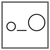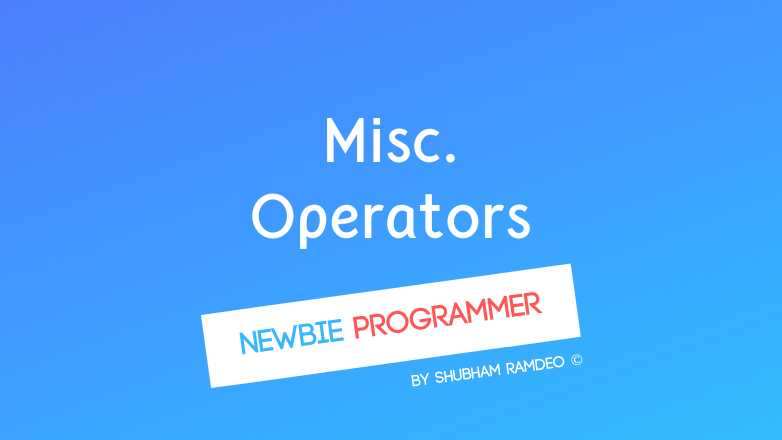Fuddlepixel coz tech is fun();

# Miscellaneous Operators and Operator Precedence

Let us discuss the some important miscellaneous operators available in C language to carry some important stuffs and also the operator precedence.

By Shubham Ramdeo
11 September 2015 · 8 mins read
# `c`  `newbie`Some extra about operator to carry some important stuffs beautifully !

Welcome to Newbie Programmer Series. In the last post we have got an introduction to what bitwise operators are. In this “last” post of operators series, we will learn some Miscellaneous operators and then the precedence of operators in C. If you are new to this series, please go to index (click here) and read out all the previous parts so that you can easily understand what I am talking about.

This is going to be a long post I think. Anyways, Please Continue reading below :

## Miscellaneous Operators

In C, we got some useful miscellaneous operators that help us to perform some important tasks. We are just beginner at this level so I won’t be going too deep into them but present a brief introduction.

### sizeof()

This operator tells us the size of the variable. Just like all the files on a computer have some storage size like 2 MB, 1 GB etc, variables are too stored into computer’s memory. And with this sizeof() operator we can know how much memory they will require so that we can choose some different ways of programming to make our program faster.

USAGE : sizeof ( variable name )

So if you write int a = 3;

then printf(“%d”, sizeof( a ) );

will show you the size of variable a on the screen.

### Address ( & )

When we store a variable in memory, it is stored in a particular space. Just like when we keep our money in bank, we get a specific bank Account Number. Similarly, variables are too stored at a specific address and we can know it by using the “&” operator.

USAGE : &variable name

It’s actually too simple. Suppose if you have defined  variable int a = 3;

Then printf(“%d”, &a);

Will show you the Variable’s account Number

### Pointer ( * )

If you are in a bank, you know your account number, your account size, money you have but you don’t know where you account is kept. Pointer can tell you the direction : HERE !. Pointer knows where a variable is kept. It gives the direction to the address where the variable is kept. It does not have any value. It will simply point towards the variable.

USAGE : *****variable name

So if you define int a = 3;

then *a will direct you to variable a.

### Example Diagram

I find it hard to understand so I have made a labelled diagram for you guys so that you can easily understand the meaning of above operators.### Conditional Operator ( ? )

Well we will later learn how to add conditions in a program using “if - else”, this conditional operator ( ? ) works the same way. With this operator we can add a condition which needs to be true to perform a certain task. Other wise, the other task we be performed.

USAGE : (condition to be true)? (to do if true): (to do otherwise)

Lets do a great example below :

``````#include <stdio.h>
main()
{
int num;
printf("Enter the Number : ");
scanf("%d",&num);
(num%2==0)?printf("Even"):printf("Odd");
}
``````

What this program is doing that it get a number from you and finds its reminder on dividing by 2. If the reminder is 0 it prints “Even”. Otherwise “Odd”.

## Operator Precedence in C

Have you ever heard of DMAS in mathematics ? Suppose you want to solve x = 3 + 10 / 5 - 1 * 4. What will you do first ? Multiply Or divide or what you like the most, that one ? Obviously NOT. In such case we have to follow this DMAS rule. Which says First divide, them multiply then add and subtract at last. So the x = 1. In C, we not only have these one but also a lot of other operators. So we have to follow a rule similar to DMAS in maths. The following table below is that one. As in DMAS, we have DIVISION with highest precedence, The operator at the top of this table also has the highest precedence and will be performed first. And the last one is of lowest precedence and will be formed at the end.  So the table below is organized in decreasing order of precedence.

Also we have to follow the direction of operators. If I say 10/5, you will say 2 and not 0.5 because we follow division Left to Right. Hence the table below also contains the direction of the operators.

And don’t worry. I know it’s not easy to get all this now in brain because we have not performed any of them practically. We will learn them in time after doing a lot of practical applications of these operators.

 Postfix () [] -> . ++ - - Left to right Unary + - ! ~ ++ - - (type)* & sizeof Right to left Multiplicative * / % Left to right Additive + - Left to right Shift << >> Left to right Relational < <= > >= Left to right Equality == != Left to right Bitwise AND & Left to right Bitwise XOR ^ Left to right Bitwise OR | Left to right Logical AND && Left to right Logical OR || Left to right Conditional ?: Right to left Assignment = += -= *= /= %=>>= <<= &= ^= |= Right to left Comma , Left to right

AND HERE ENDS THE OPERATORS ! I am so glad. Actually bored of it. Anyways, In the next part, we will start something new and interesting and that will require a lot of coding. So be Excited and Stay Connected.

Any doubts ? Meet me at twitter @ramdeoshubham

##### Update me weekly ✉

Subscribe below and we’ll send you a weekly email summary of all new Code tutorials. Never miss out on learning about the next big thing.

#### Shubham RamdeoShubham Ramdeo is the founder of Fuddlepixel. He is a geek, a writer, involved with many technical and humanity projects.

Meet me!

#### Recent#### Related

`c`

`newbie`

Top